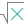#SUMSQ (PQL - xl)

This function returns the sum of the squares of the arguments

• Library: PQL \ Spreadsheet \ Math & Trig
• Compatibility: Any content (regardless of data source) in the Tabulate spreadsheet module
• Solve: This function can be used with the Solve plug-in: both as part of the objective function and constraint functions

#### Syntax

SUMSQ(number1, number2)

##### Function Arguments
 Name Description Type Optional number1 Double-precision floating-point value, an integer value, or an array (cell range) of these StringOrNumber number2 Double-precision floating-point value, an integer value, or an array (cell range) of these Number